• 易语言分数运算模块源码,分数运算模块,分数运算,分数赋值,带分数赋值,求分数次方,求分数平方根,约分,带分数约分,通分,分数负号移分子,带分数负号移分子,文本到分数,文本到带分数,分数到文本,带分数到文本,数值到分数...
• 分数运算模块解方程计算器源码系统结构:分数运算,求分数次方,求分数平方根,约分,带分数约分,通分,分数负号移分子,带分数负号移分子,文本到分数,文本到带分数,分数到文本,带分数到
• 相加运算，该运算接收两个分数对象的分子和分母，然后进行分数相加，结果保存在自己的分子和分母中，运算规则为：假设当前分数为A0/B0，接收两个分数A1/B1和A2/B2，相加结果，A0=A1×B2 + A2×B1，B0=B1×B2 ...
题目描述 用C++定义和实现一个分数类，并根据要求完成分数对象的运用
分数类包含分子和分母两个属性，操作包括：
各属性的get方法  构造函数，初始化分子分母  相加运算，该运算接收两个分数对象的分子和分母，然后进行分数相加，结果保存在自己的分子和分母中，运算规则为：假设当前分数为A0/B0，接收两个分数A1/B1和A2/B2，相加结果，A0=A1×B2 + A2×B1，B0=B1×B2  相乘运算，该运算接收两个分数对象的分子和分母，然后进行分数相乘，运算规则为：假设当前分数为A0/B0，接收两个分数A1/B1和A2/B2，然后进行分数相乘，A0=A1×A2，B0= B1×B2  显示函数，显示格式：分数/分母
在主函数中定义若干个分数对象，并使用分数对象进行运算。
提示：每个分数运算都包含三个分数对象，例如要做分数A和B的相加，那么除了A和B还要定义C，通过调用C的相加方法，把A和B的分子分母传递给C运算，结果保存在C中
注意：提交代码时必须用注释划分出三个区域：类定义、类实现、主函数，如下
//-----类定义------ class XXX
{ // 写类定义代码
};
//----类实现------
void Process::XXX()
{ // 写类定义代码
};
//-----主函数-----
int main()
{
//写对象的创建和运行过程
return 0;
}
输入 第一行输入t表示有t个测试实例
第二行输入第一个分数的分子和分母，都用整数输入，都不为0
第三行输入第二个分数的分子和分母，都用整数输入，都不为0
依次类推输入其他实例的分数分子和分母
注意：如果分数是负数，负号在分子中输入，例如负的五分之三，则分子为-3，分母为5
输出 输出每个实例的运算结果，每个实例包含三行
第一行输出要运算的两个分数对象
第二行输出两个分数相加的结果，注意相加的运算、结果显示都要使用第三个对象的方法
第三行输出两个分数相乘的结果，注意相乘的运算、结果显示都要使用第三个对象的方法
样例输入 2 2 3 4 5 -3 4 5 6 样例输出 2/3 4/5 22/15 8/15 -3/4 5/6 2/24 -15/24
#include <iostream>
#include<iomanip>
#include<cmath>

using namespace std;

//-----类定义------
class CFraction
{
private:
int fz, fm;
public:
CFraction();
CFraction(int fz_val, int fm_val);
void add(const CFraction& a, const CFraction& b);
void mul(const CFraction& a, const CFraction& b);
int getFz();
int getFm();
void print();
};

//----类实现------
CFraction::CFraction()
{
fz = 0;
fm = 0;
}
CFraction::CFraction(int fz_val, int fm_val)
{
fz = fz_val;
fm = fm_val;
}
int CFraction::getFz()
{
return fz;
}
int CFraction::getFm()
{
return fm;
}
void CFraction::add(const CFraction& a, const CFraction& b)
{
fz = a.fz * b.fm + b.fz * a.fm;
fm = a.fm * b.fm;
}
void CFraction::mul(const CFraction& a, const CFraction& b)
{
fz = a.fz * b.fz;
fm = a.fm * b.fm;
}
void CFraction::print()
{
cout << fz << "/" << fm;
}

//-----主函数-----
int main()
{
int fz1, fm1, fz2, fm2;

int t;
cin >> t;
while (t--)
{
cin >> fz1 >> fm1;
cin >> fz2 >> fm2;
CFraction c1(fz1, fm1), c2(fz2, fm2);
CFraction c3;
c1.print();
cout << " ";
c2.print();
cout << endl;
c3.print();
cout << endl;
c3.mul(c1, c2);
c3.print();
cout << endl;
}
return 0;
}


展开全文c++
• ## C语言 分数运算

千次阅读 2019-11-04 22:46:56
题目 读入4个整数a、b、c、d和一个运算符（‘+’、‘-’、‘*’、‘/’中的一个），进行分数a/b和c/d的对应运算，输出最简结果。...对应每组测试数据，输出分数运算的最简结果，占一行。具体可参照样例...
题目
读入4个整数a、b、c、d和一个运算符（‘+’、‘-’、‘*’、‘/’中的一个），进行分数a/b和c/d的对应运算，输出最简结果。
输入
有多组测试数据。输入的第一行是整数T(1<=T<=200)，表示随后测试数据的组数。每组测试数据占一行，由一个四个正整数a,b,c,d和一个运算符组成，相邻数据间有一个空格
输出
对应每组测试数据，输出分数运算的最简结果，占一行。具体可参照样例。
样例输入 Copy
3 1 2 3 4 - 35 24 24 5 * 25 72 9 5 /
样例输出 Copy
1/2-3/4=-1/4 35/24*24/5=7 25/72/9/5=125/648
解题思路
#include<stdio.h>
#include<math.h>
int main(){
int n,a,b,c,d,e,fz,fm,m,i,j,p,f=1,l;/*n：几组数据；fz：分子；fm:分母；f:1正0负；*/
scanf("%d",&n);
for(i=1;i<=n;i++){/*for循环*/
scanf("%d %d %d %d %c",&a,&b,&c,&d,&e);
f=1;
{
if(e=='-'){fz=a*d-b*c;fm=b*d;}
if(e=='+'){fz=a*d+b*c;fm=b*d;}
if(e=='/'){fz=a*d;fm=b*c;}
if(e=='*'){fz=a*c;fm=b*d;}   /*根据数学计算分别赋值分子分母*/
}
if(fz%fm==0)          /*整除即输出*/
printf("%d/%d%c%d/%d=%d\n",a,b,e,c,d,fz/fm);
else{
if(fz<0){f=0;fz=-fz;}/*分子为负时f赋0*/
if(fz>fm)p=fm;
else p=fz;/*p存储分子分母较小值*/
for(j=2;j<=p;j++){
for(l=2;l<=j;l++)
if(fz%l==0&&fm%l==0){
fz=fz/l;fm=fm/l;}
}/*约分分子分母*/
if(f==1)printf("%d/%d%c%d/%d=%d/%d\n",a,b,e,c,d,fz,fm);
else printf("%d/%d%c%d/%d=%d/%d\n",a,b,e,c,d,-fz,fm);  /*根据f值分情况输出*/
}
}

}

展开全文C语言
• 分数运算 时间限制 1000 ms 内存限制 32768 KB 代码长度限制 100 KB 判断程序 Standard (来自 小小) 题目描述 计算机中采用浮点数表示所有实数，但这意味着精度丢失。例如无法精确表示“1/3”。 ...
分数运算
时间限制 1000 ms 内存限制 32768 KB 代码长度限制 100 KB 判断程序 Standard (来自 小小)
题目描述
计算机中采用浮点数表示所有实数，但这意味着精度丢失。例如无法精确表示“1/3”。
NowCoder最近要处理很多金融问题，这些账目不允许出现精度丢失，因为差之毫厘谬之千里。你能帮他实现一套分数的计算器吗？

输入描述:
输入包含多组数据。
每组数据一行，包含两个分数和一个运算符，中间用空格隔开。
其中分子与分母均为不大于30的正整数。

输出描述:
对应每一组数据，输出两个分数的计算结果。
要求输出最简分数，即分子与分母互质。

输入例子:
1/3 2/3 +
1/5 1/4 -
1/2 1/3 *
2/3 4/3 /

输出例子:
1/1
-1/20
1/6
1/2
#include<stdio.h>
int gcd(int a,int b)
{
while(b!=0)
{
int r=b;
b=a%b;
a=r;
}
return a;
}
int main()
{
int a,b,c,d,s1,s2,x;
char y;
while(scanf("%d/%d%d/%d %c",&a,&b,&c,&d,&y)!=EOF)
{
if(y=='+')
{
s1=a*d+b*c;
s2=b*d;
}
if(y=='-')
{
s1=a*d-b*c;
s2=b*d;
}
if(y=='*')
{
s1=a*c;
s2=b*d;
}
if(y=='/')
{
s1=a*d;
s2=b*c;
}
x=gcd(s1,s2);
s1=s1/x;
s2=s2/x;
if(s1<0&&s2>0)
printf("-%d/%d\n",-s1,s2);
else if(s1>0&&s2<0)
printf("-%d/%d\n",s1,-s2);
else if(s1<0&&s2<0)
printf("%d/%d\n",-s1,-s2);
else
printf("%d/%d\n",s1,s2);
}
return 0;
} 

展开全文• 声明：原文链接C++(标准库)分数运算（ratio库） 一、ratio概述 自C++11起，C++标准库提供了一个接口允许你具体指定编译期分数，并允许对它们执行编译期运算 以下摘自[N266:Chrnon]并略有改动： 二、ratio的实现 ...
声明：原文链接  C++(标准库)分数运算（ratio库）
一、ratio概述
自C++11起，C++标准库提供了一个接口允许你具体指定编译期分数，并允许对它们执行编译期运算
以下摘自[N266:Chrnon]并略有改动：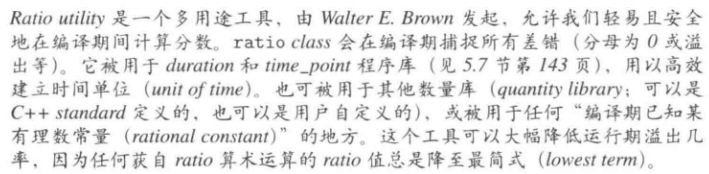二、ratio的实现
ratio由<ratio>提供，其定义如下：
type：产出相应类型，其也是一个ratio<>，其返回自身，可以直接使用域访问父访问num：分子den：分母，默认为1
其所有成员都是public的
intmax_t是个带正负号的整数类型，其有能力表现出任何带正负号的整数，其被设计于<cstdint>或<stdint.h>内，至少具备64bit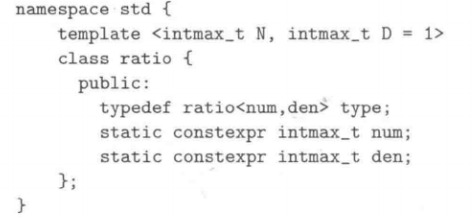演示案例
int main()
{
typedef ratio<5, 3> FiveThirds;
std::cout << FiveThirds::num << "/" << FiveThirds::den << std::endl;

typedef ratio<25, 15> AlsoFiveThirds;
std::cout << AlsoFiveThirds::num << "/" << AlsoFiveThirds::den << std::endl;

typedef ratio<42,42> one;
std::cout << one::num << "/" << one::den << std::endl;

typedef ratio<0> zero;        //分母默认为1
std::cout << zero::num << "/" << zero::den << std::endl;

typedef ratio<7, -3> Neg1;    //-号会被移动到分子身上
std::cout << Neg1::num << "/" << Neg1::den << std::endl;

typedef ratio<-7, 3> Neg2;    //分子的-号保持不变
std::cout << Neg2::num << "/" << Neg2::den << std::endl;

typedef ratio<-7, -3> Neg3;   //分子分母的-号都会消失
std::cout << Neg3::num << "/" << Neg3::den << std::endl;
}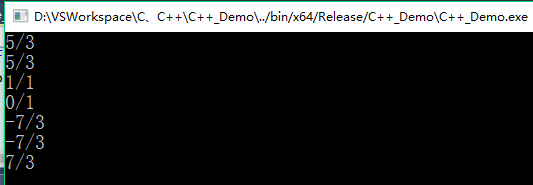三、ratio提供的操作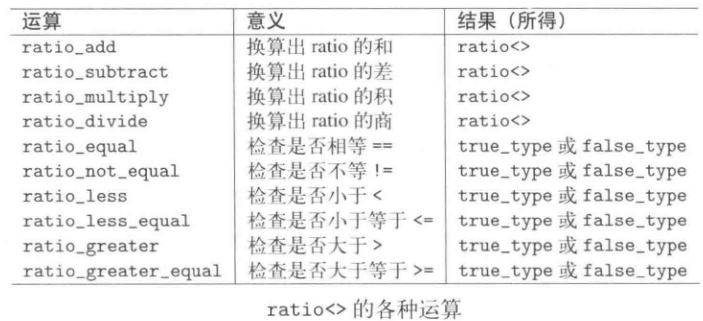基本运算符
type返回的一个是ratio<>，2/7+2/6=13/21
typedef std::ratio_add<std::ratio<2, 7>, std::ratio<2, 6>>::type test;

std::cout << test::num << "/" << test::den << std::endl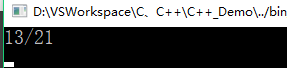分数比较

其他的一些操作用来比较两个ratio类型。它们的返回值也是true_type或false_type，其成员value返回true或false（true_type、false_type在前面文章介绍过：https://blog.csdn.net/qq_41453285/article/details/105454812）演示案例：
std::cout << boolalpha;
std::cout << std::ratio_equal<std::ratio<1, 3>, std::ratio<1, 3>>::value << std::endl;
std::cout << std::ratio_equal<std::ratio<2, 3>, std::ratio<1, 3>>::value << std::endl;
std::cout << std::ratio_greater<std::ratio<2, 3>, std::ratio<1, 3>>::value << std::endl;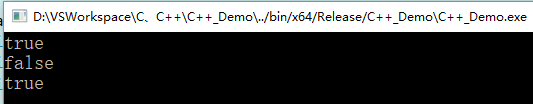四、使用ratio的一些注意事项
关于分母的一些注意事项：
ratio定义时，其分母不能定义为0，否则编译不通过ratio定义时，如果不填分母，默认为1关于负号（-）的一些注意事项：
如果分子无-号，分母有-号，分母的-号会被移动到分子身上如果分子有-号，分母无-号，则分子的-号保持不动如果分子分母都有-号，则-号都被消除
分子可以为0ratio会自动对分子和分母进行约分
演示案例
ratio会捕捉所有差错，例如分母为0、溢出等等。因此下面的无法编译通过
//无法编译通过，因为1/max乘以1/2会导致溢出，分母超过了其类型所能涵盖的极限
std::ratio_multiply<std::ratio<1, std::numeric_limits<long long>::max()>, std::ratio<1, 2>>::type;
与上面同理，下面的表达式也无法通过编译，因为其除以了0：
typedef ratio<5, 3> FiveThirds;
typedef ratio<0> zero;          //分子为0，分母默认为1
std::ratio_divide<FiveThirds, zero>::type;
但是下面的可以编译通过，因为下面只使用了ratio_divide<>，却没有实例化一个ratio<>对象，因此编译器检测不出来
typedef ratio<5, 3> FiveThirds;
typedef ratio<0> zero;
std::ratio_divide<FiveThirds, zero>; //只调用了ratio_divide，却没有实例化出任何ratio对象
五、预定义的ratio单位
ratio程序库预先定义好了很多ratio单位，可以直接使用。如下图所示：
下图中的“optional”代表，只有“当它们可被intmax_t表达时”才被定义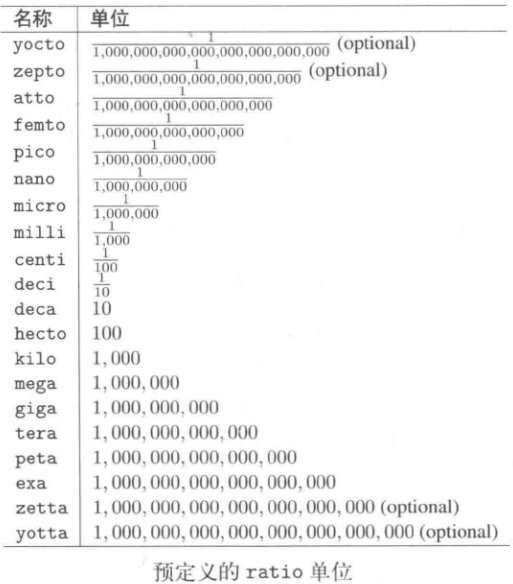演示案例：
typedef std::ratio<1, 1000000000LL> test;
std::cout << test::num << "/" << test::den << std::endl;

typedef std::nano _nano;
std::cout << _nano::num << "/" << _nano::den << std::endl;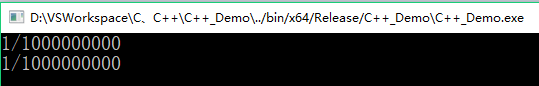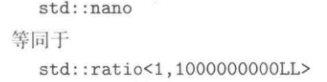这个演示案例也使得我们可以比较方便的指出纳秒
展开全文• 相加运算，该运算接收两个分数对象的分子和分母，然后进行分数相加，结果保存在自己的分子和分母中，运算规则为：假设当前分数为A0/B0，接收两个分数A1/B1和A2/B2，相加结果，A0=A1×B2+ A2×B1，B0=B1×B2 相乘运算...c++
• } else if (this->up > this->down) { //假分数:分子>分母 printf("%lld %lld/%lld", this->up / this->down, abs(this->up) % abs(this->down), this->down); } else { //真分数:分子up, this->down); } printf("\...
• ## python进行分数运算

千次阅读 2020-04-29 14:08:07
python中用于分数计算的模块是fractions，本篇博客内容来自官网：fractions — 分数 文章目录一、fraction的基本用法二、如何避免浮点数（float）和有理数转化“失真”1、float类型引号引起来2、limit_denominator...python
• golang分数运算相关函数 支持精准运算 支持加减乘除 支持链式表达式 支持结果输出(flat64) 调用示例 e 1、 加法 tmp := fractional.Model(7, 12) tmp1 := fractional.Model(1, 12) fmt.Println(tmp.Add(*tmp1)) ...
• 3. 相加运算，该运算接收两个分数对象的分子和分母，然后进行分数相加，结果保存在自己的分子和分母中，运算规则为：假设当前分数为A0/B0，接收两个分数A1/B1和A2/B2，相加结果，A0=A1×B2 + A2×B1，B0=B1...
• 受Tim老师启发,将概率的显示改成了分数,以避免精度问题.以下只实现了乘法与加法,减法和除法可以类推. 1functionFraction(numerator,denominator){2//分数类3this.n=numerator;4this.d=denominator;5}67...
• 分数至11/13 Java类，用于分数的除法，乘法，余数，加法和减法。Java
•易语言模块源码
• PostgreSQL精确分数有效的自定义类型。 非常适合精确的算术运算或用户指定的表行排序。 拥有与整数一样大的值，并且分母具有匹配的精度。 为PostgreSQL提供精确的分数有效的自定义类型。 非常适合精确的算术运算或...
• 本文为博主原创文章，未经博主允许不得转载。 //score.h #include<iostream> #include<...class score //分数类模板 { private: T molecular; //分子 T denominator; //分母 pubc++
• 编程语言：C++ 编译环境：VS2008 实现功能：分数运算的重载 参考方向：C++ 操作符重载 适用于初学者参考
• 通过类的形式，实现了分数的约分以及分数的加减乘除四则运算...